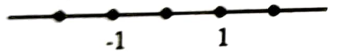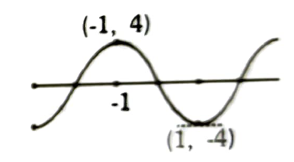Theory of expressions
Question

# Let  be given by $\mathrm{f}\left(\mathrm{x}\right)={\mathrm{x}}^{5}-5\mathrm{x}+\mathrm{a}$ then,

Difficult
Solution

## Let $\mathrm{y}={\mathrm{x}}^{5}-5\mathrm{x}$ (ii) Also, at x = 0, y = 0, thus the curve passes through the origin.        $=5\left(\mathrm{x}-1\right)\left(\mathrm{x}+1\right)\left({\mathrm{x}}^{2}+1\right)$Now,  thus f(x) is increasing in these intervalAlso, $\frac{\mathrm{dy}}{\mathrm{dx}}<0\left(-1,1\right)$ thus decreasing in (-1, 1).(iv) Also , at x = -1,  $\frac{\mathrm{dy}}{\mathrm{dx}}$ changes its sign from + ve to vex= - 1 is point of local maxima.Similarly, x = 1 is point of local minimaLocal maximum value,$\mathrm{y}=\left(-1{\right)}^{5}-5\left(-1\right)=4$Local minimum value,$\mathrm{y}=\left(1{\right)}^{5}-5\left(1\right)=-4$Now, let y = -aAs, evident from the graph, if Then, f(x) has three real roots and if  then f(x) has one real root.

Get Instant Solutions